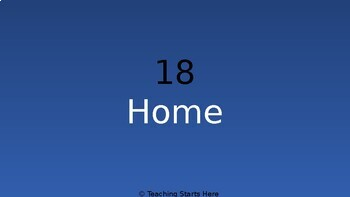# Multiplication Interactive Game Show: word problems, fact fluency and fill inSubject
Resource Type
File Type

Presentation (Powerpoint) File

(225 KB|63 pages)
Product Rating
Standards
• Product Description
• StandardsNEW

This interactive product follows the same style as Jeopardy. It covers multiple third grade standards and gives students the opportunity to review their facts, solve word problems and fill in the blanks. It is intended to be shown on PowerPoint.

During the presentation, a dollar amount can be selected. When the dollar amount is selected the question will pop up. Once the students solve the question, you can click on theanswer. This will bring you to the correct answer. The page with the answer has a button to link back to the first page or home.

The categories are:

Facts 1-5

Facts 6-10

Word Problems

Arrays

Fill in the blank

The preview includes Facts 1-5 100 point question. Please try to preview before purchasing.

Interpret a multiplication equation as a comparison, e.g., interpret 35 = 5 × 7 as a statement that 35 is 5 times as many as 7 and 7 times as many as 5. Represent verbal statements of multiplicative comparisons as multiplication equations.
Determine the unknown whole number in a multiplication or division equation relating three whole numbers. For example, determine the unknown number that makes the equation true in each of the equations 8 × ? = 48, 5 = __ ÷ 3, 6 × 6 = ?.
Use multiplication and division within 100 to solve word problems in situations involving equal groups, arrays, and measurement quantities, e.g., by using drawings and equations with a symbol for the unknown number to represent the problem.
Interpret products of whole numbers, e.g., interpret 5 × 7 as the total number of objects in 5 groups of 7 objects each. For example, describe a context in which a total number of objects can be expressed as 5 × 7.
Total Pages
63 pages
Included
Teaching Duration
30 minutes
Report this Resource to TpT
Reported resources will be reviewed by our team. Report this resource to let us know if this resource violates TpT’s content guidelines.